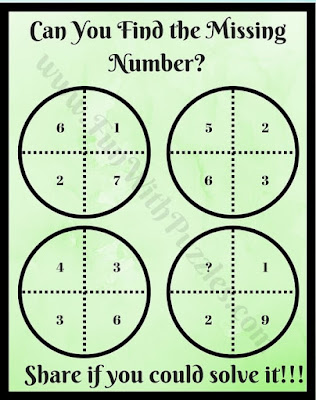Can you find the missing Numbers in the Circles? There are many different types of Math Puzzles that can be created using Circle. For Example, there are Math Circle Puzzles in the circle that is divided into different segments with each segment is containing a number. These numbers have some logical relationship with other numbers around them and one has to find this relationship and then solve it for the given segment where the number is missing. There are also Circle Math Logic Puzzles where five numbers around the circle are given. Four outside the circle and one is inside the circle. Again one has to find the relationship between these numbers and then solve it for the missing number in one of the circles. Today's Circle puzzles are an extension of these puzzles.
In each of these Math Puzzle Questions, there are four circles containing four numbers. These numbers in each circle have some mathematical or logical relationship with each other. One has to find this relationship among these numbers and then has to calculate the missing number in one of the circles?
Answers to these puzzles are given at the end of this post. Puzzles here are arranged in the order of their difficulty with the first one being the easiest one and the last one being the tough puzzle. Let's see how many of these puzzles can you solve quickly?1. Can you find the missing number?2. Brain Challenge to find the missing number3. Can you calculate the value of the missing number?4. Can you crack this puzzle by finding a missing number?5. What is the value of the missing number?

Do checkout below mentioned Maths Brain Teasers and Puzzles to exercise your brain
List of Brain Challenging Brain Teasers and Puzzles

1. Rapidly Maths Puzzles for Kids with Answers: Here are some of the easy Math Puzzles Questions for kids which need to be answered very quickly.

2. Easy Maths Puzzles Brain Teasers for Kids with Answers: It contains another set of Easy Maths Puzzles which is designed for school-going students.

3. Maths Logic Puzzles Brain Teasers with Answers: Do checkout these Maths Puzzles which will not only test your Maths skills but also your logical reasoning.

4. Brain Twister Mathematics Puzzles: After practicing all the easy and medium level Maths Puzzles, now it's time to twist your brain with these brain-twisting puzzles.

Answers of Missing Number Math Circle Puzzles
Answers along with explanations of the first 4 Maths Circle Puzzles are given below. We have held the answer to the last 5th Puzzle for the readers. Do post answers to the last puzzle in the comments. To understand the answers and explanation of these awesome missing numbers Maths Circle Puzzles let's mark each segment of Circle with A, B, C, D starting from the top left segment and then moving clockwise.

1.
Explanation: C=A+B+D

2.
Explanation: A+B = C+D

3.
Explanation: A+C=B+D

4.
Explanation: A x C = B x D

Unknown said...

HOW

Rajesh Kumar said...

You can find the answers to these missing number math circle puzzles on this page. Please let me know if you have any issue reading these answers?

sundar said...

a + b = c + d -2
so missing # is 8